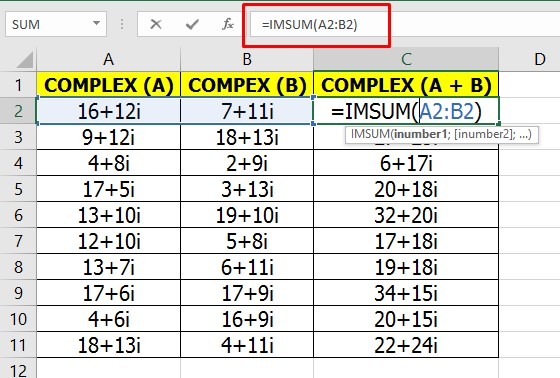# How to ADD Complex Numbers in Excel

How to ADD Complex Numbers in Excel. Complex numbers are numbers that consist of real and imaginary numbers. Complex numbers are widely used in explaining problems in the field of physics, such as explaining the wave equation (Schrodinger's equation). Complex number operations are different from ordinary addition, in that these equations have real and imaginary parts. To add two complex numbers, we must add the real and imaginary parts separately. You have to add the real part with the other real part, as well as the imaginary part.

The process of adding complex numbers manually is very easy. As I explained above, that is adding up the two parts separately. However, if you want to add complex numbers in excel, then you need to know the complex number function in excel and how to write complex number formulas in excel. On this occasion, I will discuss how to add two complex numbers in Excel using the Excel complex function.

## How to ADD Complex Numbers in Excel

How, to add two complex numbers in Excel? You can use the function IMSUM(number1;number2;number3;...). Currently, I use excel 2016 the separator between numbers is (;) for other versions of excel sometimes use (,) to separate one number from another, such as IMSUM(number1,number2,number3,...).

Important: How to write excel numbers in excel.

=COMPLEX(Real Number; Imaginary Number)

=COMPLEX(A1;B1)

=COMPLEX(2;3) we get 2 + 3i

Number1 is the first complex number and Number2 is the second complex number, and so on. So, if you have two complex numbers, the addition function is IMSUM(number1;number2).

Suppose you have two complex numbers namely A = 2 + 3i and B = 3 + 2i. What is the sum of the two complex numbers and what is the value of the sum of the two numbers?

1. The first step, please open the Microsoft Excel application.
2. Write the complex numbers A = 2 + 3i and B = 3 + 2i. Suppose complex number A is written in cell A1 and complex number B is written in cell B1.
3. The function for adding these two complex numbers in excel is =IMSUM(A1; B1).
4. Then the sum of the two complex numbers is 5 + 5i.### Modulus or Magnitude of Complex Number in Excel

To calculate the magnitude of a complex number in excel is almost the same as calculating the magnitude of a vector. Where the real number is the X-axis and the imaginary number is the Y-axis, then the magnitude of the complex number is the root(x^2 + y^2). How to separate real and imaginary numbers in excel. To retrieve real values in excel, please use the IMREAL(inumber) function. As for the imaginary value, please use the IMAGINARY(inumber) function.

1. Modulus or Magnitude of Complex Number in Excel
2. =SQRT((IMREAL(inumber)^2)+IMAGINARY(inumber)^2)
3. inumber is a complex number. Suppose the complex number is in cell C2 then the magnitude function in excel:
4. =SQRT((IMREAL(C2)^2)+IMAGINARY(C2)^2)